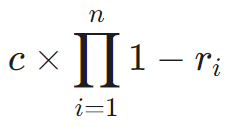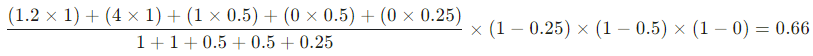# Risk engine

The risk engine in Arculix by SecureAuth is responsible for calculating the level of assurance (LOA) for each transaction. LOA will be used by the smart MFA module to decide whether to increase or decrease the friction for the user.

The risk engine in Arculix comes with an out of the box integration with the Policy engine.

## Risk analyzers

Risk analyzers are responsible for fetching data from different sources and calculating a final score based on the collected information. Each risk analyzer focuses on a specific area and gets a specific kind of data. For example, the IP risk analyzer gets some information about the IP address of the user and generates a score based on that address.

This is a non-inclusive list of built-in risk analyzers:

• AIML: Provides a score based on the user contextual information provided to the AI/ML engine in Arculix.

• Auth method: Provides a score based on the authenticator used for the last MFA.

• DBFP: Provides a score based on the user's browser fingerprint.

• IP: Provides a score based on the user's IP address.

• Location: Provides a score based on the user's obtained location. The location will be obtained from the phone or browser and if not provided, falls back to the IP-based location.

## Third-party risk analyzers

The risk analyzers are designed to be modular and there are multiple ways of integrating new risk analyzers:

• Calling an external API to fetch the score for a given user and context

• An external risk engine can call the generic score API to provide the score for the users

• A custom risk analyzer module can be implemented by the SecureAuth team that can communicate with any external resources

## LOA score

The LOA score is a number between 0.0 to 4.0. The higher the LOA the more likely the user is who they claim to be. The overall LOA score is calculated out of the confidence and risk scores generated by the Risk analyzers.

### Total LOA score calculation

The calculation happens in two steps.

#### 1. Total confidence score

We collect the confidence scores reported by the risk analyzers. Then, we calculate the total confidence score as a weighted average of individual confidence scores and their weights.

#### 2. Total LOA score

We collect the risk scores reported by risk analyzers and define the Reversed risk score as one minus the risk score value (1 - risk_score) for each risk score. To calculate the Total LOA score, we multiply the Total confidence score from the previous section by the Reversed risk score of each risk analyzer:Where:

• $c$ is the Total confidence score from the previous step

• $n$ is the total number of risk scores

• ${r}_{i}$ is the risk score from the ${i}^{th}$ risk analyzer

#### Example

Consider the following risk analyzers information.

Risk analyzer

Confidence score

Risk score

Weight

Notes

DBFP

(Device browser fingerprint)

1.2

N/A

1

Auth Method

4

N/A

1

Because OOB method was push

IP

1

N/A

0.5

GPS

0

N/A

0.5

Because location data is not available (e.g. user didn't allow permission)

AnalyzerX

0

N/A

0.25

Third-party risk analyzer

ThreatSignal

N/A

0.25

N/A

ThreatSignal service recognized a risk

AnalyzerY

N/A

0.5

N/A

AnalyzerZ

N/A

0

N/A

No risk

The total LOA score is equal to: